# Data Compression (Ch 7)¶

### Huffman Tutorial

This expands on the Shortest Symbol trick from Nine Algorithms and introduces a concrete algorithm for coming up with a shortest symbol code called Huffman encoding.

 We want to compress messages that only consist of the characters: a, b, c, d, and e. They are used in the proportions: 3 a’s, 1 b, 2 c’s, 1 d and 3 e’s. First, write them down in order from most common to least common.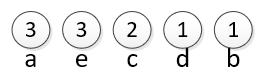We take the least common pair and join them. The new circle has a value of 2 because it represents the combined d and b (1 of each).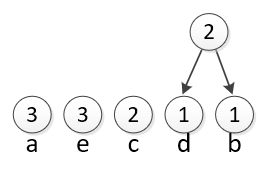Now we do it again to join the smallest two ungrouped circles together. The new circle has a 4 because it represents 2 c’s, and the group of 2 d’s and b’s.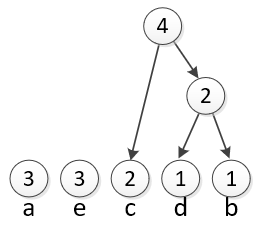Again, join the smallest two remaining circles. The two smallest circles are the a and e (3 instances of each). So we will join them into a group of 6. You always merge the smallest circles. Do not just work from right to left!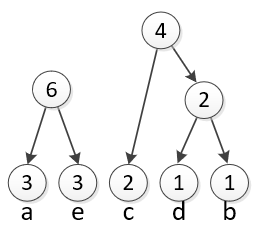Finally, we can merge the two remaining circles into one final circle. Once we are left with just one group, we are ready to make the code.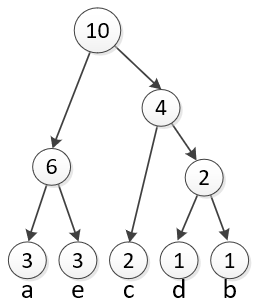Think of each circle as choosing between two branches. Put a 0 on the left branch and a 1 on the right. To get the code for a letter, read off the 0’s and 1’s along the branches you take to get from the top circle to the given letter. ‘a’ would be coded as 00 ’d’ would be coded as 110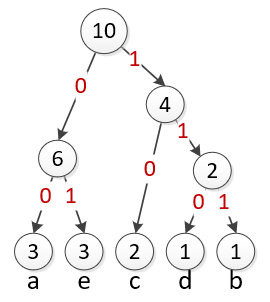The full table of codes is shown in the table below. Note that the process of building the code resulted in two important features: 1) The most common symbols have the shortest codes. 2) No code is a prefix of another code.

 a 00 e 01 c 10 d 110 b 111

Using the code, the sequence aabcea is encoded as “00 00 111 10 01 00”. But because of the way we designed the code, we don’t even need the spaces. We could decode “0000111100100” by working our way from left to right. as shown below:

Code

Decoded

Notes

0000111100100

Start

0000111100100

Is 0 a code? No, continue…

0000111100100

a

Is 00 a code? Yes, it represents a. We have used up the 00.

0000111100100

a

Is 0 a code? No, continue…

0000111100100

aa

Is 00 a code? Yes, it is another a. We have used up that 00.

0000111100100

aa

Is 1 a code? No, continue…

0000111100100

aa

Is 11 a code? No, continue…

0000111100100

aab

Is 111 a code? Yes, it represents b. We have used up the 111.

0000111100100

aab

Is 1 a code? No, continue…

0000111100100

aabc

Is 10 a code? Yes, it represents c. We have used up the 10.

0000111100100

aabc

Is 0 a code? No, continue…

0000111100100

aabce

Is 01 a code? Yes, it represents e. We have used up the 01.

0000111100100

aabce

Is 0 a code? No, continue…

0000111100100

aabcea

Is 00 a code? Yes, it represents a. We have used up the 00.

0000111100100

aabcea

We have consumed all the bits, the message was aabcea